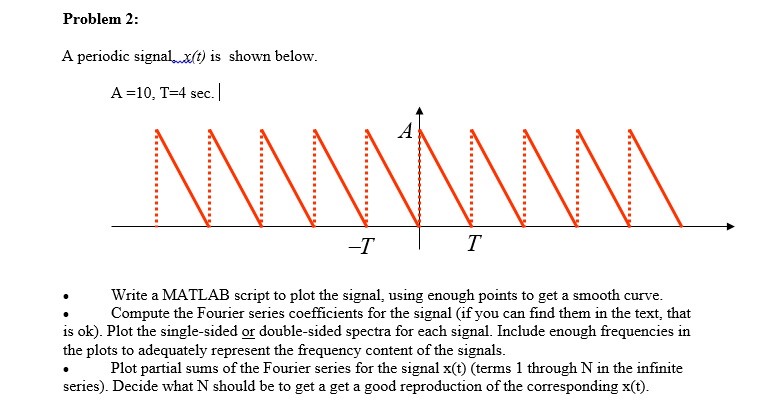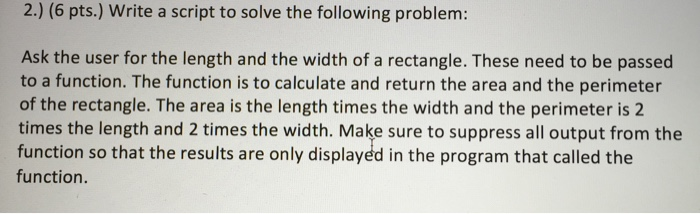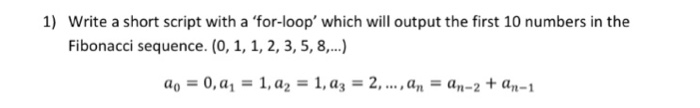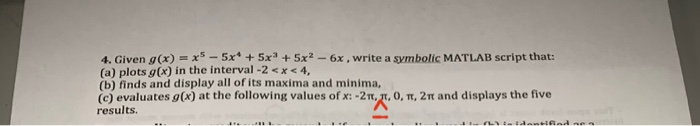Question

Write a Matlab script to generate random sequence of 10 bits using AMI, Manchester techniques and draw 2 plots. The third plot will display the combination of AMI and Manchester data.

We need at least 10 more requests to produce the answer.

0 / 10 have requested this problem solution

The more requests, the faster the answer.

All students who have requested the answer will be notified once they are available.

#### Earn Coins

Coins can be redeemed for fabulous gifts.

Similar Homework Help Questions
• ### In matlab script, thank you! Write a MATLAB script that will generate random integers in the...In matlab script, thank you! Write a MATLAB script that will generate random integers in the range from 1 to 100, and print them, until one is finally generated that is greater than 50. The script should print how many attempts it took

• ### Solving digital system problems using MATLAB Write a MATLAB script CTask2p3.m to: a) Generate the...

Solving digital system problems using MATLAB Write a MATLAB script CTask2p3.m to: a) Generate the truth table for the following Boolean expressions and display it in the MATLAB command window (Use logical functions: and, not, or)

• ### Flowcharts & for loops 1. Using a for loop, write a MatLab script to convert ounces...

Flowcharts & for loops 1. Using a for loop, write a MatLab script to convert ounces to grams for 0 to 16 ounces, in 1-ounce increments. Present output in a table; label columns appropriately. 2. A Fibonacci sequence is composed of elements created by adding the previous 2 elements. The simplest Fibonacci sequence starts with 1, 1 and proceeds as follows: 1,1,2,3,5,8… Element 3 is the sum of elements 1 and 2 (1+1=2) Element 4 is the sum of elements...

• ### Problem 2: A periodic signalxit) is shown below A =10, T-4 sec. -T Write a MATLAB script to plot ...Problem 2: A periodic signalxit) is shown below A =10, T-4 sec. -T Write a MATLAB script to plot the signal, using enough points to get a smooth curve. Compute the Fourier series coefficients for the signal (if you can find them in the text, that is ok). Plot the single-sided or double-sided spectra for each signal. Include enough frequencies in the plots to adequately represent the frequency content of the signals. Plot partial sums of the Fourier series for...

• ### Write a matlab script(using only matlab) for this. Write a script to solve the following problem:...Write a matlab script(using only matlab) for this. Write a script to solve the following problem: Ask the user for the length and the width of a rectangle. These need to be passed to a function. The function is to calculate and return the area and the perimeter of the rectangle. The area is the length times the width and the perimeter is 2 times the length and 2 times the width. Make sure to suppress all output from the...

• ### Q: please solve using matlab code withe comment 1.      Write the Matlab code to generate: x...

Q: please solve using matlab code withe comment 1.      Write the Matlab code to generate: x = cos(w1*t) + cos(w2*t); where, w1=7; w2=23; t = [0:0.005:2] 2.   Plot the signal using sub plot a.   in time domain b.   in frequency domain 3)   Sample the signal under different sampling conditions: a.      Ts<1/2fm b.      Ts=1/2fm c.       Ts>1/2fm 4).      Multiple plot: the signal sampled under sampling condition and the signal x a.      in time domain b.      in frequency domain c.       Label and Title...

• ### By using MatLab please. Best. Write a short script with a 'for-loop' which will output the...By using MatLab please. Best. Write a short script with a 'for-loop' which will output the first 10 numbers in the Fibonacci sequence. (0, 1, 1, 2, 3, 5, 8, ...) a_0 = 0, a_1 = 1, a_2 = 1, a_3 = 2, ..., a_n = a_n-2 + a_n-1

• ### Write a MATLAB script to generate a 512 × 512 test image consisting of a white...

Write a MATLAB script to generate a 512 × 512 test image consisting of a white circle against a black background, use this image as an input to the fft2 function, and display the resulting spectrum. You should be able to notice the presence of “ringing” artifacts in the resulting spectrum, owing to the sharp transitions between the circle and the background. Andimplement the solution to the ringing artifact, while keeping the input image similar to the original (white circle...

• ### 4. Given (x)=x-5x + 5x5x2 - 6x , write a symbolic MATLAB script that: (a) plots...4. Given (x)=x-5x + 5x5x2 - 6x , write a symbolic MATLAB script that: (a) plots g(x) in the interval -2 <x< 4, (b) finds and display all of its maxima and minima, (c) evaluates g(x) at the following values of x: -21, 21, 0, 1, 2st and displays the five results. tidad

• ### Using MATLAB, generate a sequence of L=4000 independent Gaussian random variable with variance σ^2 = N0/2. MATLAB code to generate one Gaussian random variable with variance σ^2: \sqrt(σ^2)∗randn. Mak...

Using MATLAB, generate a sequence of L=4000 independent Gaussian random variable with variance σ^2 = N0/2. MATLAB code to generate one Gaussian random variable with variance σ^2: \sqrt(σ^2)∗randn. Make sure that N0 has to be set to a proper value, depending on the value Eb/N0 (dB) you’re working on.